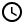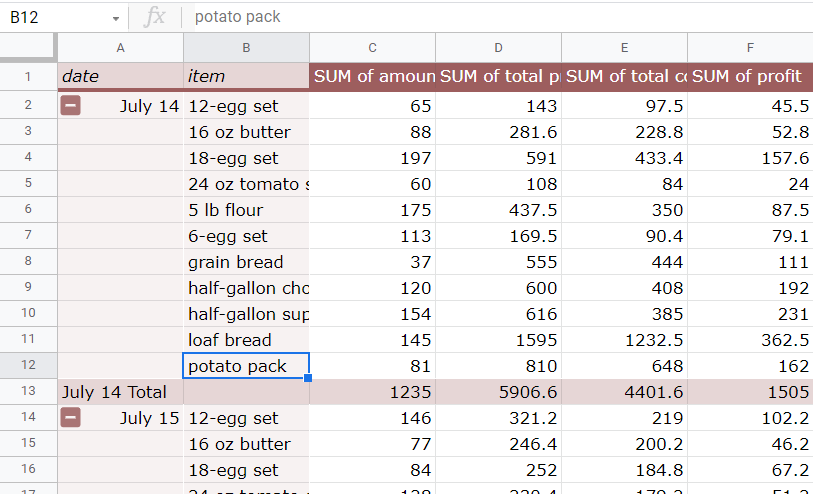How to Add Two-Tier Row Labels to the Pivot Table in Google Sheets

Learn how add two-tier row labels to pivot tables in Google Sheets.1. How to add the second-tier row labels to the Pivot Table
2. How to rearrange the hierarchy of the row labels
3. How to hide the second-tier row labels5 Minutes

Let’s say we have the following raw data:

And we want to summarize this into a Pivot Table. You can arrive at the following:

If you look back at the raw data, there are two columns that can be used to organize the pivot table: the item and the date. The item column was used in the first Pivot Table to summarize the data. The second column, the date column, can also be added as the second row header column to the Pivot Table. In this tutorial, we will learn how to achieve this:

How to add the second-tier row labels to the Pivot Table

Here are the steps to add the second-tier row label as the second column in the Pivot Table:

Step 1: Click on any cell in the Pivot Table so that the Pivot table editor sidebar appears on the right side of Google Sheets.

As you can see, the item column is used as the row labels or headers in the Pivot table.

Step 2: Click Add button on the Rows header in the Pivot table editor sidebar.

A drop-down box of the list of columns will appear. Select the column you want to use. For our case, we want to select the date column.

The final Pivot Table can be seen below:

How to rearrange the hierarchy of the row label tiers

In our original two-tier row labels Pivot Table, the item names are at the first level while the dates are at the second level. As you might have figured out, it will make more sense if the dates are set as the first level label while the item names are at the second level. To do so, go to the list of Rows on the Pivot table editor sidebar, then drag the Dates box up, flipping the order. The result is shown below.Pivot Table two-tier row labels, with the date now being the first row labels and the item names being the second row labels.

How to hide the second-tier row labels

Sometimes you need to temporarily hide the second-tier row labels. To do so, simply click the small box with the minus sign below the first level row label:

The result is:

Oops! Something went wrong while submitting the form.

December 26, 2021

How to Add Two-Tier Row Labels to the Pivot Table in Google SheetsLet’s say we have the following raw data:

And we want to summarize this into a Pivot Table. You can arrive at the following:

If you look back at the raw data, there are two columns that can be used to organize the pivot table: the item and the date. The item column was used in the first Pivot Table to summarize the data. The second column, the date column, can also be added as the second row header column to the Pivot Table. In this tutorial, we will learn how to achieve this:

How to add the second-tier row labels to the Pivot Table

Here are the steps to add the second-tier row label as the second column in the Pivot Table:

Step 1: Click on any cell in the Pivot Table so that the Pivot table editor sidebar appears on the right side of Google Sheets.

As you can see, the item column is used as the row labels or headers in the Pivot table.

Step 2: Click Add button on the Rows header in the Pivot table editor sidebar.

A drop-down box of the list of columns will appear. Select the column you want to use. For our case, we want to select the date column.

The final Pivot Table can be seen below:

How to rearrange the hierarchy of the row label tiers

In our original two-tier row labels Pivot Table, the item names are at the first level while the dates are at the second level. As you might have figured out, it will make more sense if the dates are set as the first level label while the item names are at the second level. To do so, go to the list of Rows on the Pivot table editor sidebar, then drag the Dates box up, flipping the order. The result is shown below.Pivot Table two-tier row labels, with the date now being the first row labels and the item names being the second row labels.

How to hide the second-tier row labels

Sometimes you need to temporarily hide the second-tier row labels. To do so, simply click the small box with the minus sign below the first level row label: